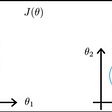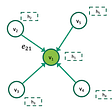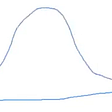# A Smooth Introduction to Linear Regression and its Implementation in PyTorch (Part-II)

So in Part-I I gave a simple introduction on what linear regression is and how we can find the equation of the best fit line for our data. In this post, I will show you how to implement the task we worked on in Part-I in PyTorch. So, let’s get started!

The first step is to import the libraries we will be using:

`import torchimport torch.nn as nnimport numpy as npimport matplotlib.pyplot as plt`

Now, we need to set the hyperparameters, as follows:

`input_size = 1output_size = 1epochs = 50learning_rate = 0.0001`

The input size is set to 1 since our inputs to the model are scalars h (hour in the day). The output is also set to 1 since we will get only one value returned for r (number of pages being read). So, basically, we will leave our program to find the best values for B_0 and B_1 that we calculated in the previous part of this tutorial.

Let’s now enter the training data, which will represent the values of h and r that we already have.

`h_train = np.array([,,,,,],dtype=np.float32)r_train = np.array([,,,,,],dtype=np.float32)`

To perform linear regression we have to define three things: model (linear regression), loss function, and the optimizer, after which we can take our data to training. The figure below depicts this process.

Let’s take that step-by-step in PyTorch. So, first we define our linear regression model:

`model = nn.Linear(input_size,output_size)`

Then, define the loss function (mean squared error):

`criterion = nn.MSELoss()`

And the optimizer (stochastic gradient descent):

`optimizer = torch.optim.SGD(model.parameters(),lr=learning_rate)`

We can now train our model with the number of epochs specified (i.e. 5).

`# train the modelfor epoch in range(epochs):	inputs = torch.from_numpy(h_train)	targets = torch.from_numpy(r_train)	# forward propagation	predictions = model(inputs)	loss = loss_function(predictions,targets)	# backward propagation	loss.backward()	# optimization	optimizer.step()`

Oh, to get an idea on what’s going on after each epoch, we can add the following statement in our training for-loop:

`print 'Epoch: ' + str(epoch) + '.....' + 'Loss: ' + str(loss.item())`

That’s it! You have just written a PyTorch program that will find the best fit line (i.e. linear regression) for our data which describes how many pages the person read at each hour of the day.

Let’s go ahead and plot our best fit line against the original data we provided the model with, as follows:

`prediction = model(torch.from_numpy(h_train)).detach().numpy()plt.plot(h_train, r_train, 'v--g', label='original data')plt.plot(h_train, prediction, 'r', label='best fit line')plt.legend()plt.show()`

And,….. this is how our best fit line looks like!

If you would like the full code, you can kindly find it here.

--

--

--

## More from Dr. Abder-Rahman Ali

Leveraging machine/deep learning and image processing in medical image analysis | https://abder.me

Love podcasts or audiobooks? Learn on the go with our new app.

## Automatic Text Summarization with Machine Learning — An overview## Leveraging Power of Reinforcement learning in Digital Marketing## Introduction To Natural Language Processing## How To Create A Perfect Decision Tree?## Feature Scaling: Why and How?## Mastering Random Forests: A comprehensive guide## Leverage Graph Neural Networks (GNNs) to assist with Regulatory & Compliance based use cases for…## Spelling Error Correction## Dr. Abder-Rahman Ali

Leveraging machine/deep learning and image processing in medical image analysis | https://abder.me

## Using Random Forests to help explain why MLB players make the Hall of…## Introduction to Linear Regression## Hypothesis Testing (t-tests)